﻿ Some Methods of Analysis of Chaos in Mechanical Systems

Some Methods of Analysis of Chaos in Mechanical Systems

Peter Sivák, Ingrid Delyová, Jozef Bocko, Juraj ŠarlošiOPEN ACCESSPEER-REVIEWED

Some Methods of Analysis of Chaos in Mechanical Systems

Peter Sivák1,, Ingrid Delyová1, Jozef Bocko1, Juraj Šarloši1

1Department of Applied Mechanics and Mechatronics, Faculty of Mechanical Engineering, Technical university of Košice, Košice, Slovakia

Abstract

Solution of non-linear dynamic systems is dependent on exact knowledge of the initial conditions. Even a slight deviation of these values can cause substantial change in the overall course of the event. It then appears chaotic. Development of such dynamic system can be represented using abstract phase space through attractors, fractals, etc. A typical example is Lorenz attractor, which is in a three-dimensional view shaped as two intertwined spirals. Rössler attractor is a relatively simple system on which chaos in geometric form can be shown in a time sequence. Among the non-traditional oscillators in non-linear mechanics can be classified Duffing and Van der Pol oscillators. This paper shows an example of a chaotic attractor formed in a non-periodic mode, obtained in an experiment of water dripping from an unclosed valve.

At a glance: Figures

123456
Prev Next

• Sivák, Peter, et al. "Some Methods of Analysis of Chaos in Mechanical Systems." American Journal of Mechanical Engineering 2.7 (2014): 199-203.
• Sivák, P. , Delyová, I. , Bocko, J. , & Šarloši, J. (2014). Some Methods of Analysis of Chaos in Mechanical Systems. American Journal of Mechanical Engineering, 2(7), 199-203.
• Sivák, Peter, Ingrid Delyová, Jozef Bocko, and Juraj Šarloši. "Some Methods of Analysis of Chaos in Mechanical Systems." American Journal of Mechanical Engineering 2, no. 7 (2014): 199-203.

 Import into BibTeX Import into EndNote Import into RefMan Import into RefWorks

1. Introduction

During the study of mechanical dynamic system and the process of finding exact solutions of equations defining the dynamic system it is possible to detect whether the system will after a long time get into steady state. In this case it is possible to search for a related attractor as one of the basic tools used in chaos theory.

Development of any dynamic system can therefore be represented and described using abstract space called the phase space. If this system is left to develop, a curve or a set of points is formed in the phase space depending on whether time is continuous or discrete. If the system is allowed to develop long enough the curve in the phase space highlights a particular structure called attractor. If the attractor consists of a closed border, the system behavior can be predicted indefinitely. The chaotic behavior of a mechanical dynamic system is characterized by an attractor with unclosed border. All chaotic attractors are so called fractals. These are irregular fragmented geometric objects created using recursion. Their image after magnifying thus has the same or a very similar shape to the original image, regardless of the scale [1, 3, 4].

2. Types and Properties of Attractors

The simplest attractors are points and limit cycles. Mathematical definition of an attractor is based on the following assumptions. Let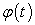be a function determining the dynamics of the system. If s is an element of phase space, i.e. parameter s completely determines the state of the system at a specific moment, then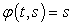and for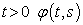develops back to s in t elements of time. Attractor is then a subset A of the phase space in such way that:

1. A is invariant to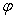, i.e. if s is element A, then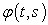also is its element for all t,

2. there is neighborhood B(A) of set A, so called region of attraction of set A, or B(A) is set of points, which in the limit t →∝enter A,

3. there is no such M with properties 1 and 2 that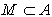and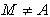.

There are several types of attractors. This may be a fixed point in case of the system stabilizing in an infinite time in any stable state, which can be calculated; e.g. a damped pendulum. Attractor can be a closed curve or a fractal. A special type of attractor is so called strange attractor, which is characterized by chaotic trajectories, for example Lorenz attractor. It describes such systems, in phase space of which two types of systems, beginning their development almost at the same initial conditions, in a very short time start to diverge and after some time will be completely different to each other.

3. Dynamic System Described by Lorenz Attractor

This attractor was first time defined by Edward N. Lorenz when he was studying a very strange dynamic system [2, 6]. It was a three-dimensional non-linear deterministic dynamic system. It related to a model for thermal convections that describe the flow of viscous liquids and also heat distribution, which is the driving force of thermal convections for example in the atmosphere. Lorenz attractor is shaped in a three-dimensional view as two intertwined spirals; it is described by a system of differential equations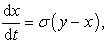(1)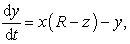(2)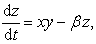(3)

where the meaning of used parameters is following: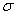- Prandtl number,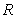- parameter proportional to Rayleigh number (reduced Rayleigh number),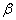- geometrical factor,

where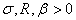.

System of differential equations, solution of which can be identified with trajectories in phase space, is an idealization of hydrodynamic systems where individual coordinates have the following meaning:

Coordinate x is proportional to intensity of convective motion, coordinate y is proportional to thermal difference between increasing and decreasing currents and coordinate z is proportional to deformation of vertical thermal profile from linearity.

Lorenz attractor is sensitive to initial conditions, what Lorenz described as “the butterfly effect“. Any small inaccuracy in terms of initial conditions leads to a large deviation from reality in the future. This effect thus implies that the behavior of complex non-linear dynamic systems is very dependent on the starting conditions and excludes any long-term predictability.

Example for parameters: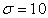,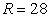,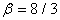Lorenz system exhibits chaotic behavior. Its trajectory in phase space is illustrated in Figure 1. For additional values of R it shows tangled periodic orbits. Also, if the value of parameter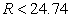, system converges to a point (Figure 2 to Figure 6).

4. Dynamic System Described by Rössler Attractor

This attractor described by Otto E. Rössler is exceptionally simple system that can easily, in geometric form, show chaos in a time sequence. It is represented by system of the following differential equations: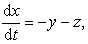(4)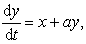(5)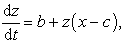(6)

System of equations with appropriate selection of input parameters a, b, c exhibits chaotic non-deterministic solution. This system also has considerable sensitivity to initial conditions. The constants a, b, c can be appropriately selected. E.g. for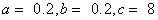Rössler attractor has shape as shown in Figure 7.

5. Duffing Oscillator

This non-linear oscillator is an example of a system that becomes chaotic during its course and this is despite being driven by a periodic force.

Duffing oscillator  can be described by differential equation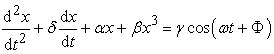(7)

or by system of differential equations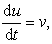(8)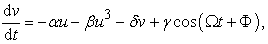(9)

where u is the displacement of x, v is the velocity and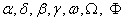are constants of the stiffness, the damping, the non-linearity in the restoring force, the amplitude and the frequency of the periodic driving force.

It is an oscillator with dual structure, where individual parts are connected with a non-stable point of equilibrium.

At steady state it is the periodic oscillations. Chaotic oscillations occur at the moment of exceeding the equilibrium point. Even Duffing oscillator, as all non-linear systems, is sensitive to a minimal change of input conditions. Using the equations that describe this oscillator its use is versatile. For example it can be used to model the flow in liquids, or predict the behavior of material strained by regular vibrations. For specific values of the parameters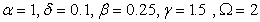it is possible to obtain attractor of Duffing oscillator according to Figure 8.

Figure 9 shows the dependence of amplitude of Duffing oscillator on time.

6. Van Der Pol Oscillator

Van der Pol oscillator with limit cycles according to Figure 10 to Figure 12 is in dynamics an untraditional oscillator with non-linear damping . It is governed by differential equation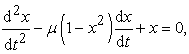(10)

where x is dynamic variable and μ > 0 is scalar parameter.

For very small x quadratic member x2 can be neglected and system thus becomes linear with negative damping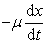.

If however x is too large quadratic member x2 becomes dominant and damping becomes positive.

Limit cycle begins as a circle and with variable value of the parameter μ gets increasingly sharper. In the case of μ = 0, damping is zero and equation (10) changes to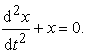(11)

7. The effect of dripping water

The first experimental study of the effect of dripping water was carried out in the university in Santa Cruz in California in collaboration of researchers Peter L. Scott, Stephen C. Pope and Philip J. Martein . The essence of one of the experiments was the water droplets from unclosed or leaking valve hitting a microphone. Measured were the time intervals between sound impulses caused by the dripping droplets.

The experiment yielded also the following findings. In the case of harmonic impact of the droplets the time differences between individual impacts are equal and in state space and create a closed cycle. In this case, it is not a chaotic course of events. Chaotic attractor can only arise in the non-periodic mode of water dripping from a valve. Random impact of droplets is caused by invisible influences, such as small vibrations or air currents. In that case the intervals are unrelated and applying the data to the coordinate system, randomly placed points are generated.

Dependence of the number of droplets on time intervals between their individual impacts can look like shown in Figure 13. Visualisation of time differences between droplets [ti+0, ti+1], [ti+1, ti+2] for 2D view and time differences [ti+0, ti+1, ti+2], [ti+1, ti+2, ti+3] for 3D view then yields dependencies according to Figure 14 to Figure 17.

Figure 17. Distribution of time intervals between individual impacts in 3D space in logarithmic coordinates

8. Conclusion

Laws of dynamics allow accurately predicting the course of physical events and creating deterministic models, if driving forces and initial conditions are known with sufficient precision. Purely deterministic approach is not possible to use in a whole lot of events known from the micro-world or common experience. These then become non-linear dynamic systems with non-linear properties. Such systems are very common in nature and events that in a limited period of time and at small deviation from equilibrium are perceived as deterministic can also be included among them.

Non-linear dynamic systems are described by differential equations solution of which depends on a sufficiently precise knowledge of the initial conditions. Even a slight inaccuracy or change in the values of the initial conditions results in a substantial change in the overall course of the event, which then appears as chaotic.

For chaotic dynamic systems it is impossible to predict their state after sufficiently long time period. However we find that the trajectories can with time approach subsets occupying only small part of phase space, so called attractors. Some attractors can even have fractal structure, when with gradual magnification the smaller parts “resemble” the larger ones.

Acknowledgement

This work was supported by grant projects VEGA No. 1/0393/14, VEGA No. 1/1205/12 and VEGA No. 1/0937/12.

References

  Horák, J., Krlín, L. and Raidl, A., Deterministic chaos and its physical applications, Prague, Academia 2007.In article  Lorenz, E, N., Deterministic nonperiodic flow, J. Atmos. Sci., 1963, 20.In article  Moon, F., Chaotic and Fractal Dynamics, Springer-Verlag New York, LLC. 1990.In article  Shuster, H. G.: Deterministic chaos, Physic-Verlag, 1987.In article  Luo, A., C., J. and Huang, J., "Period-3 Motions to Chaos in a Softening Duffing Oscillator" International Journal of Bifurcation Chaos, Volume 24, Issue 03, March 2014.In article  Duffing Differential Equation, [Online]. Available: http://mathworld.wolfram.com/DuffingDifferentialEquation.html. [Accessed Sept. 9, 2014].In article  Lorenz system, [Online]. Available: http://en.wikipedia.org/wiki/Chaos_theory. [Accessed Sept. 10, 2014].In article  Van der Pol Equation, [Online]. Available: http://mathworld.wolfram.com/vanderPolEquation.html. [Accessed Sept. 11, 2014].In article  Šarloši, J., Analysis of deterministic chaos in mechanical systems, Diploma thesis, Košice, 2011.In article